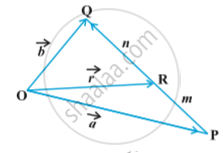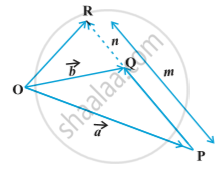# Section Formula

#### description

• Section formula for internal division
• Midpoint formula
• Section formula for external division

#### notes

Let P and Q be two points represented by the position vectors vec (OP) and vec (OQ), respectively, with respect to the origin O. Then the line segment joining the points P and Q may be divided by a third point, say R, in two ways – internally and externally. Then we find the position vector vec (OR) for the point R with respect to the origin O . We take the two cases one by one.

Case I - Fig.When R divides PQ internally . If R divides vec (PQ) such that m vec (RQ) = n vec (PR), where m and n are positive scalars, we say that the point R divides vec (PQ) internally in the ratio of m : n. Now  from triangles ORQ and OPR, we have

vec (RQ) = vec (OQ) - vec(OR) = vec b - vec r and

vec (PR) = vec (OR) - vec (OP) = vec r - vec a, Therefore we have

m(vec b - vec r) = n (vec r - vec a),

vec r = (m vec b + n vec a)/(m+n)           ( On simplification)
Hence, the position vector of the point R which divides P and Q internally in the ratio of m : n is given by
vec (OR) = (m vec b + n vec a)/(m + n)

Case II - When R divides PQ externally Fig.The position vector of the point R which divides the line segment PQ externally in the ratio m : n i.e. (PR)/(QR) = m/n is given by
vec (OR) = (m vec b - n vec a)/(m - n)

Remark:
If R is the midpoint of PQ , then m = n. And therefore, from Case I, the midpoint R of vec (PQ), will have its position vector as
vec (OR) = (vec a + vec b)/2

If you would like to contribute notes or other learning material, please submit them using the button below.

#### Video Tutorials

We have provided more than 1 series of video tutorials for some topics to help you get a better understanding of the topic.

Series 1

Series 2

### Shaalaa.com

Section Formula for Internal [00:03:33]
S
0%